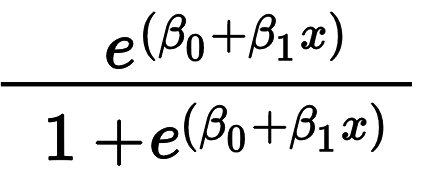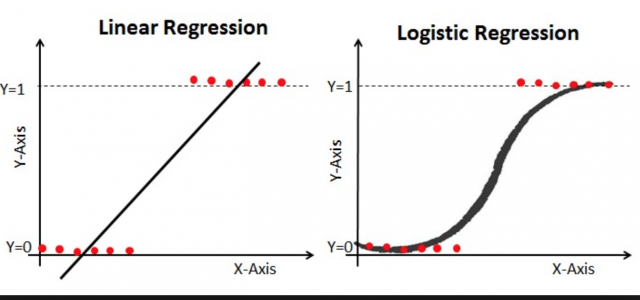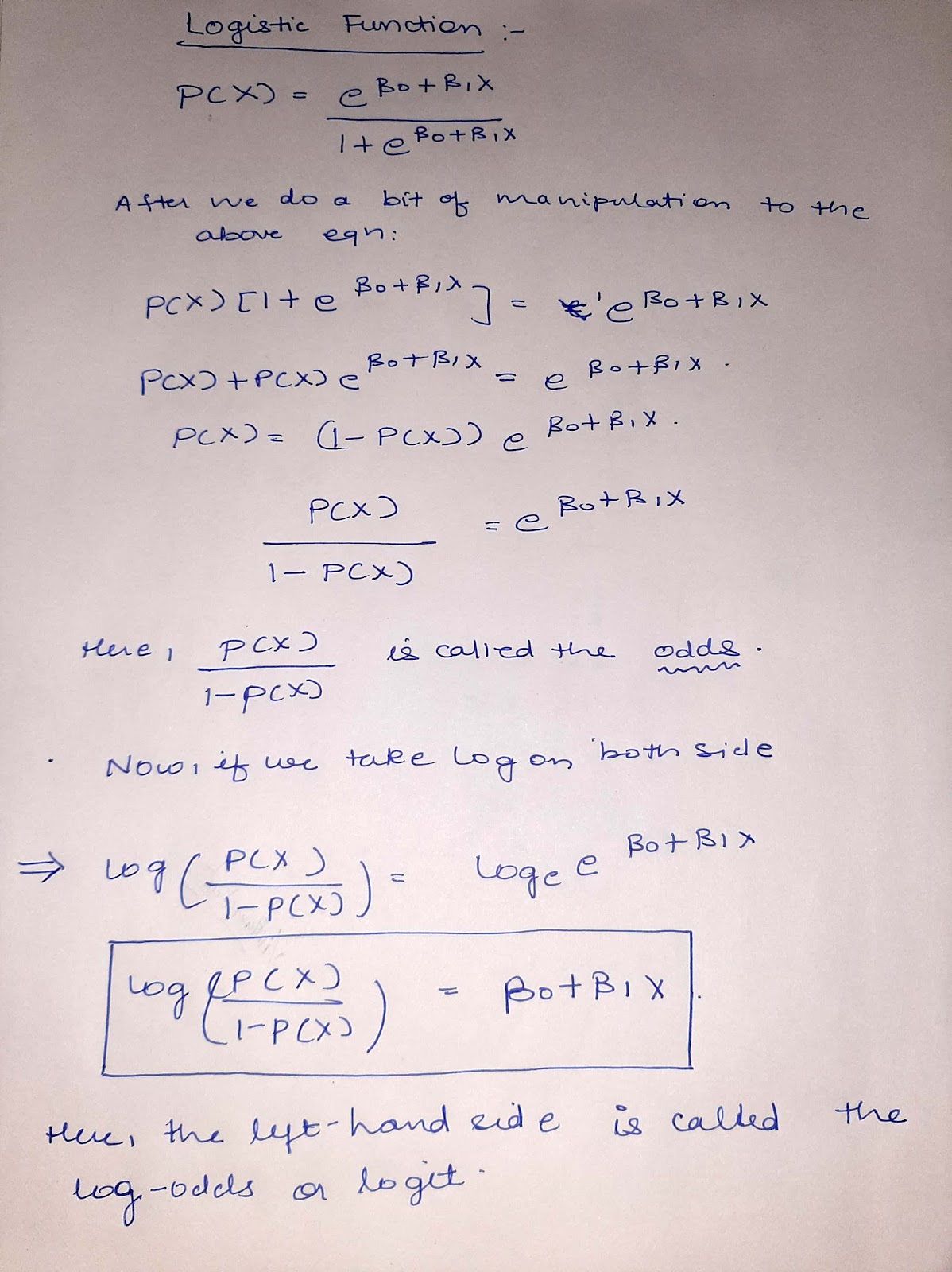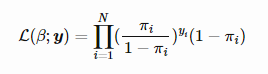# Understand the Logistic regression model in Machine Learning

Before moving on to logistic regression let's just go through some of the differences between logistic and linear regression...

Logistic regression is the big brother of linear regression. linear regression is a regression algorithm while logistic is a categorical algorithm. Linear regression works on continuous data(like weight, height, etc) whereas logistic regression works best with discrete data (like no. of persons in a class, gender of a person on the basis of height, weight, and pitch). For qualitative response linear regression is not applicable so this is where logistic regression comes into the picture.

## Introduction To Logistic Regression:

When we have only two response values like pass or fail, yes or no, true or false logistic regression models simply predict the probability of category that the response variable should belong to. Always remember that this probability ranges between 0 and 1.

## Logistic Function:The logistic regression produces an S-shaped curve in which the probability always tends to lie between 0 and 1.  Linear regression, on the other hand, fits a straight line graph.## Derivation For Logistic Function:

To explain the formula in a better way I wrote it on a paper and posted it here...## Estimating Regression Coefficient:

To estimate the regression coefficient unlike the linear regression least square method is not used, the maximum likelihood is preferred.

The maximum likelihood estimates the value of coefficients such that the observed data has a maximum probability.

## Likelihood Function:1. It is very easy to implement and simple to use.
2. Logistic regression can be used to measure the performance of other complex algorithms.
3. Provides great training efficiency.
4. Less prone to overfitting.
5. Logistic models can be updated easily using stochastic gradient descent.